torsdag 30 maj 2019

Electro-Magnetic origin of the Inertial Mass?

I would like to bring to your attention a paper by Mario Rabinowitz entitled "Electron Acceleration Gains Electron Mass Whether it Radiates or Not", which is freely available on the internet. In the paper it is shown that an accelerated charged particle produces a time varying magnetic field which in turn gives rise to an electric field that acts in opposition to the motion of the particle. The author stresses that this retardation force has hitherto not been appreciated and that it overshadows, by several orders of magnitude, any retardation force related to the energy loss due to radiation emitted by an accelerated charged particle. Let's take it again:

According to Biot-Savartz' law, a charged particle in motion gives rise to a magnetic field as illustrated below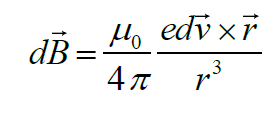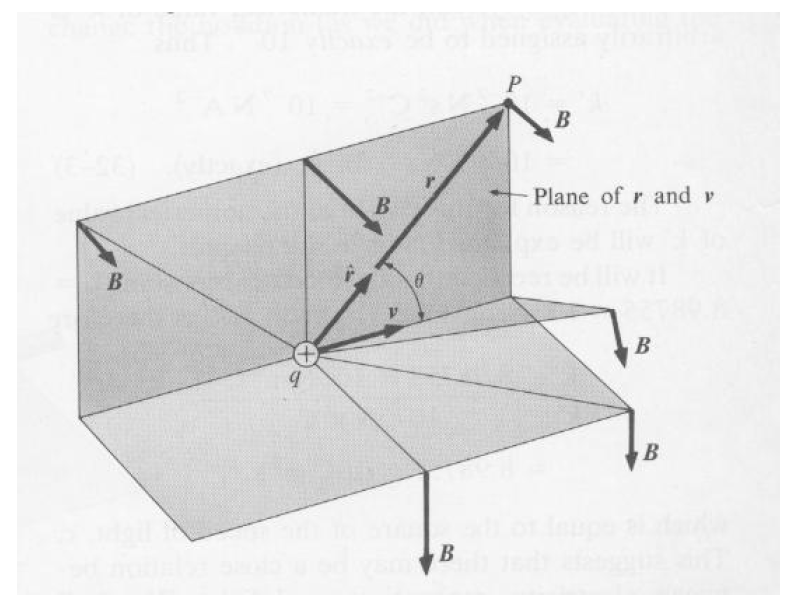where v denotes the velocity of the particle. If the particle is not only in motion, but in accelerated motion, then the induced magnetic field will be time-varying. Moreover, according to Faraday's law of induction, a time-varying magnetic field produces an electric field: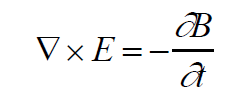It so happens that this electric field acts in direct opposition to the motion of the charged particle with a retardation force given by the following integral over infinite space: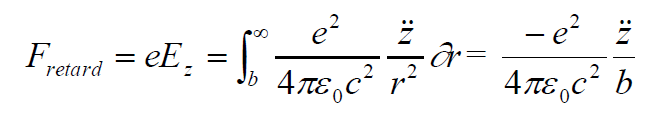Here, e is the elementary charge, c the speed of light, epsilon is the permittivity in vacuum and z with two dots on top of it is the acceleration of the particle. But there is another symbol b, what is that? It denotes the effective radius of the particle. Does an electron have a radius? you might ask, well, good question, we will get back to that soon. In sum total we arrive at the following equation for the motion of the electron: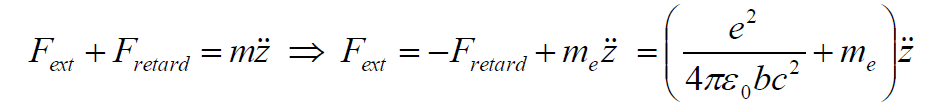Take some time to stare at these equations. What we have here essentially is Newton's second law of motion, where in addition to the ordinary inertial mass of the electron he have a contribution from its interaction with its self-inflicted electric field. But ask yourself the question, where does the inertial mass come from, has it ever been explained and not just assumed? We have been told that according to modern Quantum Electro-Dynamics, inertial mass comes from interaction with some Higgs-field that no one has ever observed outside the imagination of the CERN-scientists. But why cross the creek to get water? Why not assume that inertial mass comes from interaction with well-known fields such as the electric field. What if the inertial mass in fact IS the expression: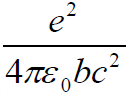But here's the catch, we have not yet evaluated the expression since it involves the hypothetical "radius" of the particle denoted by "b". If this radius is zero the expression blows up and we get infinite (!) inertial mass. Hence, let's equate the expression with the experimentally observed value for the inertial mass of the electron and see which radius we come up with. According to my calculation we end up with an electron radius of:

b = 2.81 * 10^(-15) m

which is actually quite a reasonable number given the dimensions of the atom and the nucleus that are commonly accepted. I don't know yet whether this holds up to scrutiny or is even sane, but I think it is worth a thought. If we replace the inertial mass with an expression which is dependent on the particles acceleration, there are some questions similar to "the hen and the egg" problem that enters your mind. If we give up Newton's second law in its standard form and assume that the inertial mass is not just a static property but instead viewed as a reaction to the acceleration, then what causes the acceleration in the first place? One attempt at resolution would be to say that, whenever a net external force in applied to a charged particle, it immediately tries to annihilate that net force, and the only way to do that is by accelerating.